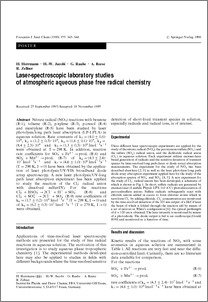### Laser-spectroscopic laboratory studies of atmospheric aqueous phase free radical chemistry

Contact
hwjacobi [ at ] awi-bremerhaven.de

Abstract

Nitrate radical (NO3) reactions with benzene (R-1), toluene (R-2), p-xylene (R-3), p-cresol (R-4) and mesitylene (R-5) have beenstudied by laser photolysis/long path laser absorption (LP-LPLA) in aqueous solution. Rate constants of k(1) = (4.0 +/- 0.6) . 10(8), k(2)= (1.2 +/- 0.3) . 10(9), k(3) = (1.6 +/- 0.1) . 10(9), k(4) = (8.4 +/- 2.3) . 10(8) and k(5) = (1.3 +/- 0.3) . 10(9) lmol(-1) s(-1) wereobtained at T = 298 K. In addition, reaction rate coefficients for SO5 + Fe2+ --> prod. (R-6) and SO5- + Mn2+ --> prod. (R-7) of k(6)= (4.3 +/- 2.4) . 10(7) lmol(-1) s(-1) and k(7) = (4.6 +/- 1.0) . 10(6) lmol(-1) s(-1) (T = 298 K, I --> 0) have been obtained by theapplication of laser photolysis/UV-VIS broadband diode array spectroscopy. A new laser photolysis/UV-long path laser absorptionexperiment has been applied to study the reaction of the Cl-2(-) radical anion with dissolved sulfur(IV). For the reactions Cl-2(-) +HSO3- --> 2Cl(-) + H+ + SO3- (R-8) and Cl-2(-) + SO32- --> 2Cl(-) + SO3- (R-9) rate coefficients of k(8) = (1.7 +/- 0.2) . 10(8)lmol(-1) s(-1) (T = 298 K, I --> 0) and of k(9) = (6.2 +/- 0.3) . 10(7) lmol(-1) s(-1) (T = 279 K, I --> 0) were obtained.

Item Type
Article
Authors
Divisions
Programs
Publication Status
Published
Eprint ID
3863
Cite as
Herrmann, H. , Jacobi, H. W. , Raabe, G. , Reese, A. and Zellner, R. (1996): Laser-spectroscopic laboratory studies of atmospheric aqueous phase free radical chemistry , Fresenius journal of analytical chemistry, 355 , pp. 343-344 .Preview
PDF (Fulltext)
Her1996c.pdf